# How to use Compute?

## How to use Compute?

Learn through detailed explanations of how to properly set up Compute conditions.

In the previous article we explained What is Compute. In this article we will explain how to set Compute.

Before making any form, survey, or quiz it would be a good idea to make a sketch, and before you make an exam or a quiz, we strongly recommend that you pay attention to the scoring and how you will deliver the results of respondent’s answers to the respondent.

Let’s take an example from previous article What is Compute!We want to make an exam or quiz and we want to add certain values or points to the answers.

With QPoint it's easy!

In the left lower corner of your question editor you have several buttons. Click on the third one which is for Compute. In the Compute dialog select "add compute", here you will set conditions to determine values for answers. For each question, you are setting up a Compute in the builder of that question. Our question is “How many stars has the American flag got”.

1. If answer - choose condition (is or is not) - is

2. Choose correct answer - 50

3. Then - choose condition (add, substract, multiply by or divide by) - Add

4. Type value or points - 1

5. To the sum of the variable - choose between predefined variables (score or price) and custom variables (you can add your variables) - Score

6. Save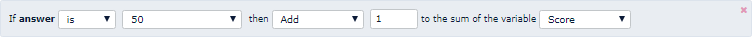This way we also set the conditions for the second question, or add one point for the correct answer.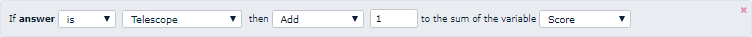Both Compute conditions: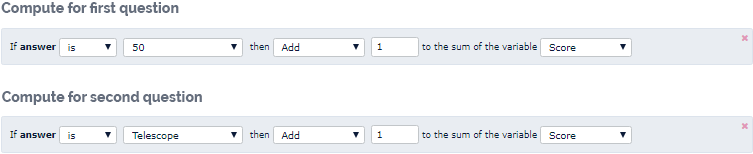In order to display the different end screens given the number of points the respondent has collected, we first add several different end screens. In our example, we put 3 different end screens.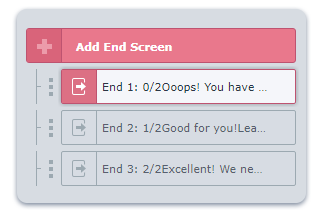The next step is to set Skip logic conditions on our last question in order to determine which end screen will be shown according to collected points.

1. If - choose from variables - Score

2. Then choose condition - is equal to

3. Type score - 0

5. Choose which end screen you want to display with according to respondent’s score - End screen 1: 0/0

We need to set up two more end screens and conditions for them, so we click on "add skip logic" for each window and set the conditions.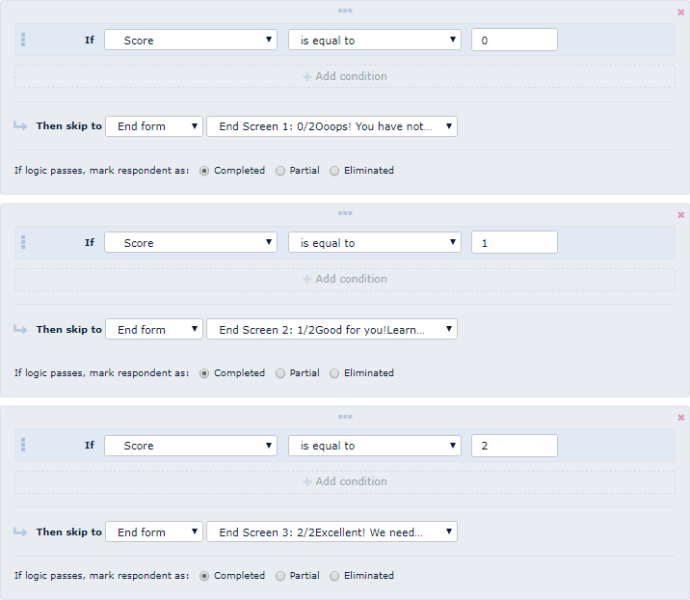Tip for exams

If you have a comprehensive exam, simply set the rating criteria according to different marks. Then, when you set Skip Logic you do not use the condition “is equal to”, but “is between” where you choose different range of points for certain marks.

Try how Compute works in our example:

Tip for quizzes

In our example, we have set a certain value only for the correct answers. When you create a quiz, each answer will probably have a certain value. Nothing terrible and unavoidable, in fact, is very easy! When you set the value for the first response in the same Compute window, select "Add Compute Action" and set the values for that response and so for all the others.

Try our personality test: